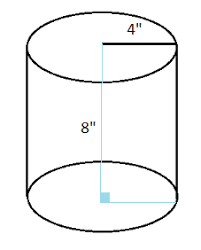# C Program Volume Of Cylinder | C Programs

C Program to find the Volume of a Cylinder – In this specific article, we will brief in on how to calculate the volume of a cylinder in C programming. The methods used to do so are as follows:

• Using Standard Method
• Using Function
• Using Pointer
• Using Macros

Suitable examples and sample programs have been added to this particular blog post for better understanding. The compiler has also been added to the post where you can execute the codes given.

As you all know, a cylinder is an important three-dimensional figure used in our lives. A cylinder is just an extension of a circle so that it is given a height of some sort.

A cylinder looks like this:As you can see, this is a cylinder with a radius of 4 inches and a height of 8 inches.

Normally, you can calculate the volume of a cylinder with this formula:

Volume = Pi * r^2 * h.

Thus, the volume of this cylinder is:

Volume = Pi * 4^2 * 8 = 402.12 sq. inch.

Thus, the methods used to calculate the volume of a cylinder in C programming are as follows:

## Using Standard Method

1)We need radius and height for calculating the volume of the cylinder.

2)The radius and height values will be stored into the variables “r”, “h”.

3)The calculated volume will store into the variable “vol”.

output:

### Using Function

1)Here we are using the float volume(float r,float h) function to calculate the volume of cylinder.

2)We are calling the function using the code v=volume(r,h).

3)The function volume(float r,float f) will calculate the volume and return the value, the return value will be assigned to variable “v”.

output:

#### Using Pointers

1)We are passing the references/addresses using volume(&r,&h,&v) to the function volume(float *r,float *h,float *a) which is having pointer variables as arguments.

2)The function volume(float *r,float *h,float *a) will calculate the volume and that value assigned to the variable pointer variable “a”

output:

##### Using Macros

1)The 2nd line represents volume(r,h) is a symbolic name to the expression (22*r*r*h)/7.

2)volume(r,h)replaced with that expression given at #define.

output:

That’s how you find or calculate the volume of cylinder C Program.

x

## C Program To Print Number Of Days In A Month | Java Tutoring

C program to input the month number and print the number of days in that ...